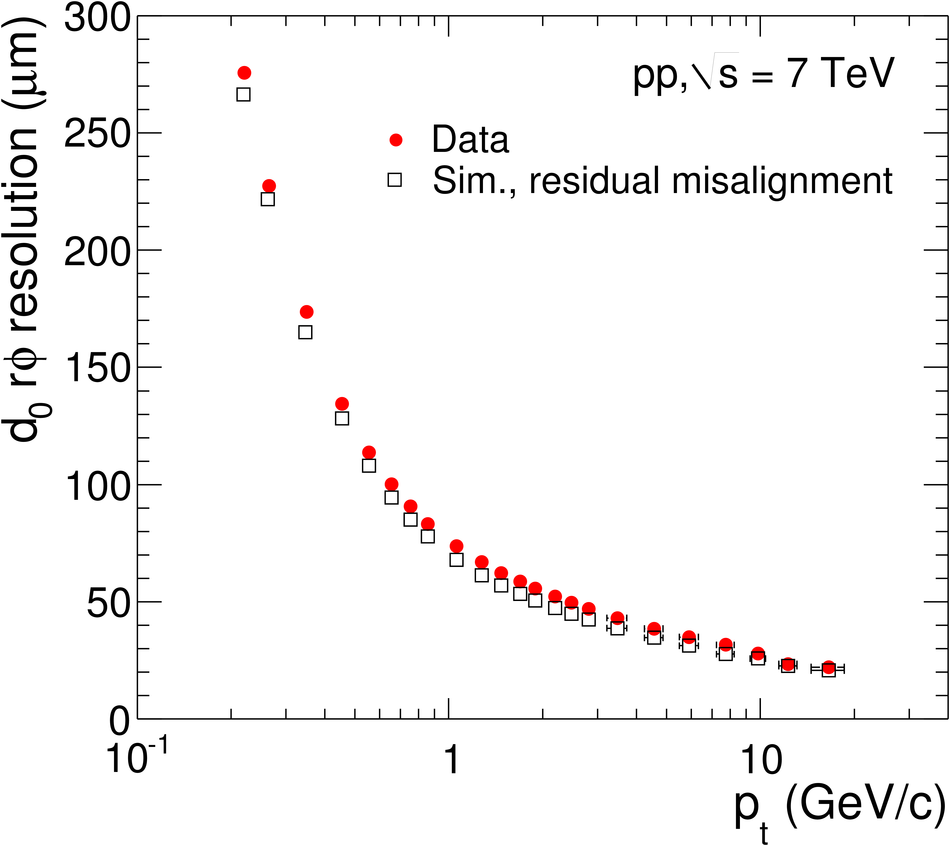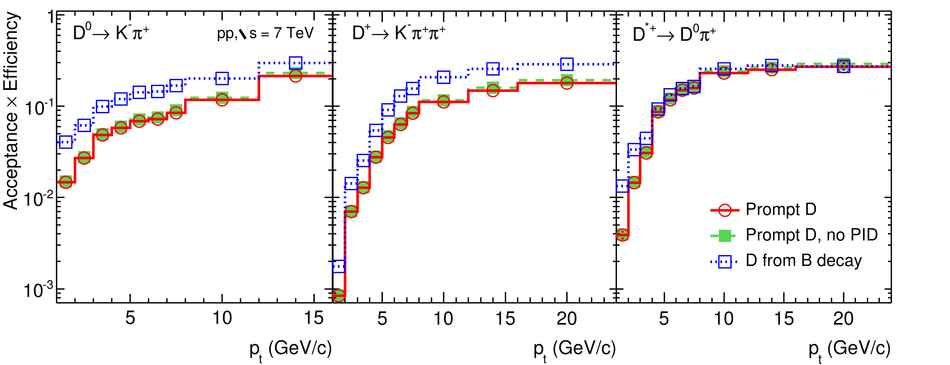Measurement of charm production at central rapidity in proton-proton collisions at $\sqrt{s} = 7$ TeV

The $p_{\rm T}$-differential inclusive production cross sections of the prompt charmed mesons $D^0$, $D^+$, and $D^{*+}$ in the rapidity range |y|<~0.5 were measured in proton-proton collisions at $\sqrt{s} = 7$ TeV at the LHC using the ALICE detector. Reconstructing the decays $D^0\rightarrow K^-\pi^+$, $D^+\rightarrow K^-\pi^+\pi^+$, $D^{*+}\rightarrow D^0\pi^+$, and their charge conjugates, about 8,400 $D^0$, 2,900 $D^+$, and 2,600 $D^{*+}$ mesons with 1<~$p_{\rm T}$<~24 GeV/$c$ were counted, after selection cuts, in a data sample of 3.14x10$^8$ events collected with a minimum-bias trigger (integrated luminosity $L_{\rm int}$ = 5/nb). The results are described within uncertainties by predictions based on perturbative QCD.

Figures

Figure 1

 Track impact parameter ($d_0$) resolution in the transverse plane($r\phi$ direction) as a function of $\pt$ comparing data and simulation. This resolution includes the uncertainty in the primary vertex position, which is reconstructed excluding the track being probed.Figure 2

 Invariant mass distributions for $\Dzero$ (top) and $\Dplus$ (middle) candidates, and mass difference distribution for $\Dstar$ candidates (bottom), for three $\pt$ intervals. The curves show the fit functions as described in the text. The values of mean ($\mu$) and width ($\sigma$) of the signal peakare reported (for $\Dzero$ and $\Dplus$ they are expressed in~$\gev/c^2$)Figure 3

 Acceptance $\times$ efficiency for $\Dzero$, $\Dplus$, and $\Dstar$ mesons, as a function of $\pt$ (see text for details).Figure 4

 Left: prompt fraction $f_{\rm prompt}$ of the $\Dzero$ raw yield as a function of $\pt$, for the two FONLL-based methods (solid: central value, from Eq.2; dashed: alternative method, from Eq.4) and for the impact parameter fit method (circles); the boxes show the envelope of the uncertainty bands of the two FONLL-based methods; the error bars show the total uncertainty from the impact parameter fit, including the statistical and systematic contributions Right: an example of $\Dzero$ meson impact parameter distribution in the transverse plane, for $2< \pt< 3~\gev/c$; the distribution is background-subtracted and fitted with the two-component function for prompt and feed-down contributions, as described in the text; the resulting prompt fraction, impact parameter resolution for prompt mesons, and $\chi^2$/(number of degrees of freedom) of the fit are given; the inset, with linear scale, shows also the negative entries, resulting from the background subtraction. \begin{align*} (2)   f_{\rm prompt}=1-(N^{\rm D^\pm~from~B~raw}/N^{\rm D^\pm~raw}) \end{align*} \begin{align*} (4)   f_{\rm prompt}= \left( 1+ \frac{({\rm Acc}\times\epsilon)_\text{feed-down} }{({\rm Acc}\times\epsilon)_{\rm prompt} } \frac{\left.\frac{{\rm d}\sigma_{\rm FONLL}^{\rm D^+~from~B}}{{\rm d}\pt}\right|_{|y|< 0.5}}{\left.\frac{{\rm d}\sigma_{\rm FONLL}^{\rm D^+}}{{\rm d}\pt}\right|_{|y|< 0.5}}\right)^{-1}\, \end{align*}Figure 5

 $\pt$-differential inclusive cross section for prompt $\Dzero$, $\Dplus$, and$\Dstar$ mesons in pp collisions at $\sqrts=7~$TeV compared with FONLL~and GM-VFNS~ theoretical predictions. The symbols are positionedhorizontally at the centre of each $\pt$ interval. The normalization uncertainty is not shown (3.5\% from the minimum-bias cross section plus the branching ratio uncertainties, as of Table 2).